# AP Physics 1 Multiple-Choice Practice Test 20

### Test Information10 questions18 minutes

1. A piano tuner needs to double the frequency of note that a particular string is playing. Should he/she tighten or loosen the string, and by what factor?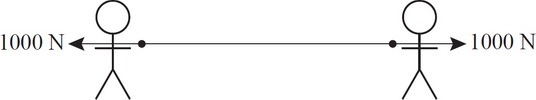2. If two people pull with a force of 1000 N each on opposite ends of a rope and neither person moves, what is the magnitude of tension in the rope?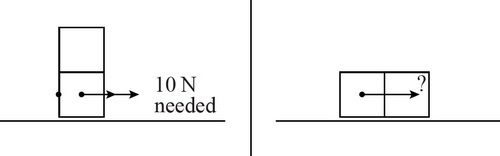3. Two identical blocks are stacked on top of each other and placed on a table. To overcome the force of static friction, a force of 10 N is required. If the blocks were placed side by side and pushed as shown in the figure above, how much force would be required to move them?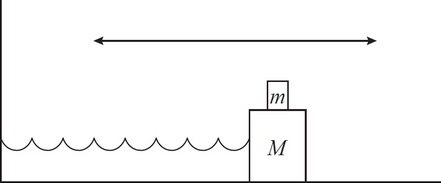4. A block of known mass M is connected to a horizontal spring that is sliding along a flat, frictionless surface. There is an additional block of known mass m resting on top of the first block. Which of the following quantities would NOT be needed to determine whether the top block will slide off the bottom block?

5. Question below refers to the below circuit diagram.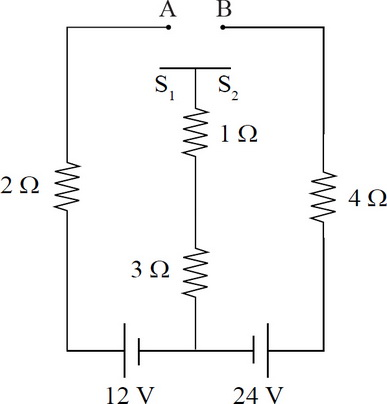If switch S1 is connected to point A but switch S2 is left unconnected, what is the current through the 1-Ω resistor?

6. Question below refers to the below circuit diagram.If switch S2 is connected to point B but switch S1 is left unconnected, what is the current through the 1-Ω resistor?

7. Question below refers to the below circuit diagram.If both switches S1 and S2 are left in the unconnected positions, what is the current through the 1-Ω resistor?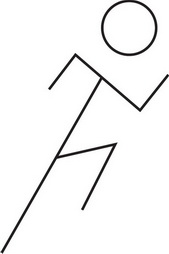8. A standing person begins to run to the right, as shown above. While the person is speeding up, which of the following could be the direction of the (total) contact force that the ground exerts on his/her foot?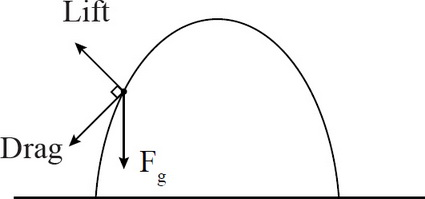9. In real projectile motion, an object experiences three forces: gravity, drag, and lift. These are depicted in the picture above. Given this information, how does lift affect the speed of a projectile?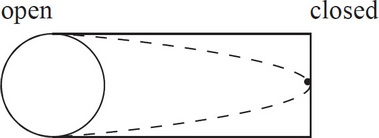10. The picture above shows a tube open at one end. A standing wave is represented by the dotted curves. If this wave has frequency f, what is the frequency of the next harmonic that can be formed in this tube?